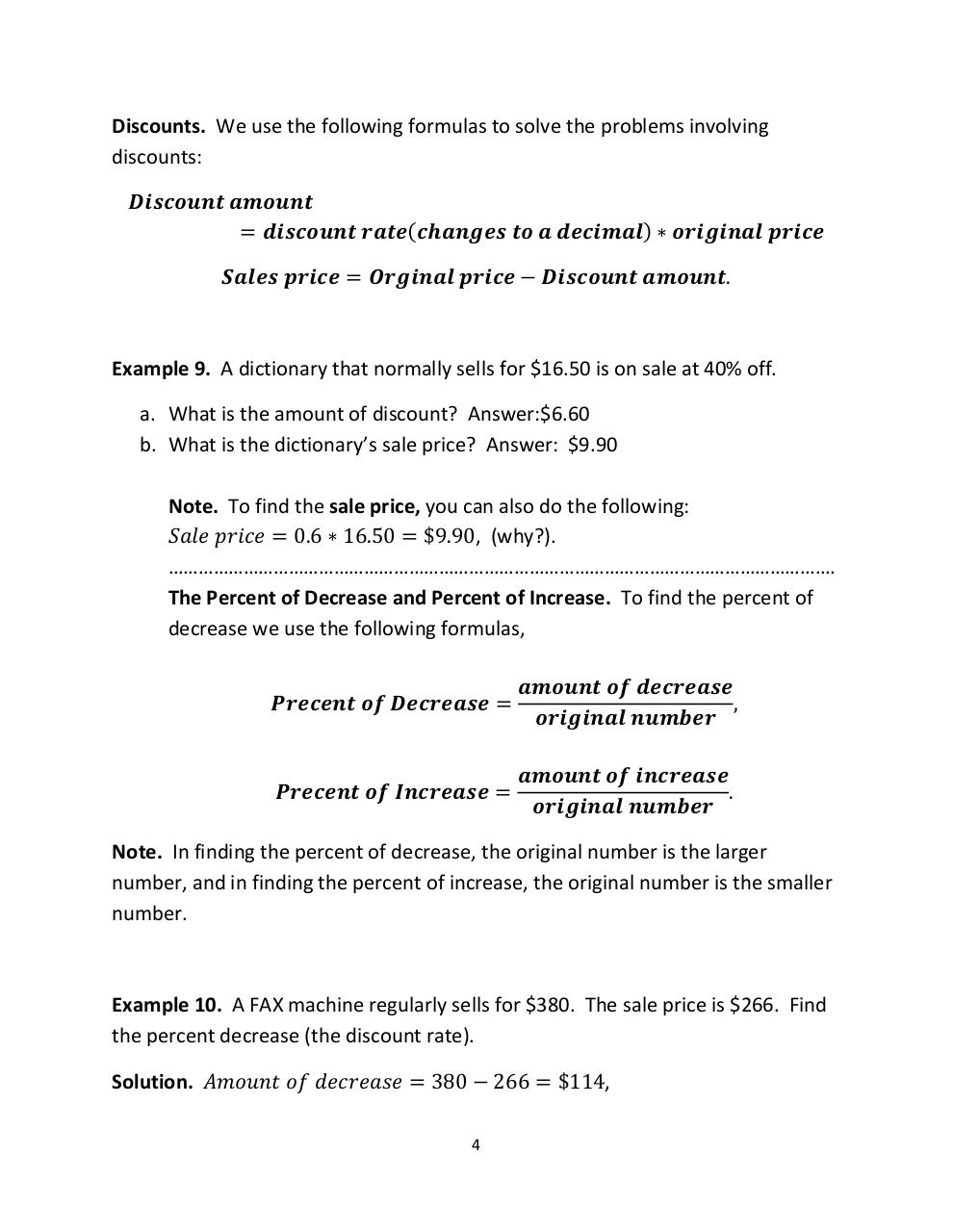# PDF Archive

Easily share your PDF documents with your contacts, on the Web and Social Networks.

## MAT143Sc8.1pdf.pdfPage 1 2 3 4 5

#### Text preview

Discounts. We use the following formulas to solve the problems involving
discounts:
𝑫𝒊𝒔𝒄𝒐𝒖𝒏𝒕 𝒂𝒎𝒐𝒖𝒏𝒕
= 𝒅𝒊𝒔𝒄𝒐𝒖𝒏𝒕 𝒓𝒂𝒕𝒆(𝒄𝒉𝒂𝒏𝒈𝒆𝒔 𝒕𝒐 𝒂 𝒅𝒆𝒄𝒊𝒎𝒂𝒍) ∗ 𝒐𝒓𝒊𝒈𝒊𝒏𝒂𝒍 𝒑𝒓𝒊𝒄𝒆
𝑺𝒂𝒍𝒆𝒔 𝒑𝒓𝒊𝒄𝒆 = 𝑶𝒓𝒈𝒊𝒏𝒂𝒍 𝒑𝒓𝒊𝒄𝒆 − 𝑫𝒊𝒔𝒄𝒐𝒖𝒏𝒕 𝒂𝒎𝒐𝒖𝒏𝒕.

Example 9. A dictionary that normally sells for \$16.50 is on sale at 40% off.
a. What is the amount of discount? Answer:\$6.60
b. What is the dictionary’s sale price? Answer: \$9.90
Note. To find the sale price, you can also do the following:
𝑆𝑎𝑙𝑒 𝑝𝑟𝑖𝑐𝑒 = 0.6 ∗ 16.50 = \$9.90, (why?).
…………………………………………………………………………………………………………………….
The Percent of Decrease and Percent of Increase. To find the percent of
decrease we use the following formulas,
𝑷𝒓𝒆𝒄𝒆𝒏𝒕 𝒐𝒇 𝑫𝒆𝒄𝒓𝒆𝒂𝒔𝒆 =

𝒂𝒎𝒐𝒖𝒏𝒕 𝒐𝒇 𝒅𝒆𝒄𝒓𝒆𝒂𝒔𝒆
,
𝒐𝒓𝒊𝒈𝒊𝒏𝒂𝒍 𝒏𝒖𝒎𝒃𝒆𝒓

𝑷𝒓𝒆𝒄𝒆𝒏𝒕 𝒐𝒇 𝑰𝒏𝒄𝒓𝒆𝒂𝒔𝒆 =

𝒂𝒎𝒐𝒖𝒏𝒕 𝒐𝒇 𝒊𝒏𝒄𝒓𝒆𝒂𝒔𝒆
.
𝒐𝒓𝒊𝒈𝒊𝒏𝒂𝒍 𝒏𝒖𝒎𝒃𝒆𝒓

Note. In finding the percent of decrease, the original number is the larger
number, and in finding the percent of increase, the original number is the smaller
number.

Example 10. A FAX machine regularly sells for \$380. The sale price is \$266. Find
the percent decrease (the discount rate).
Solution. 𝐴𝑚𝑜𝑢𝑛𝑡 𝑜𝑓 𝑑𝑒𝑐𝑟𝑒𝑎𝑠𝑒 = 380 − 266 = \$114,
4